### Aluminium Guide

UNDERSTANDING OF ALUMINUM# Warm aluminum windows

According to their strength properties, high corrosion resistance and the ability to make complex cross-sectional shape aluminum profiles It seems to be very suitable material for the manufacture of frames for windows and other different types of glazing for buildings. However, aluminum has a high thermal conductivity so, that the temperature of the window frame, which is entirely made of aluminum extrusions inside and outside the building is virtually identical, both winter, and summer.

## Warm aluminum windows

The solution to this problem is the so-called combined profiles, which is also called "warm" aluminum profiles. These profiles consist of two aluminum profiles - external and internal, which are connected with each other through a material of low thermal conductivity, such as polyamide, Polyurethane or polyvinylchloride. they say, this material with low thermal conductivity forms thermorupture, and these profiles are also called aluminum profiles with thermal.

The European standard EN 14024 and international ISO standards 10077 и ISO 15099 use the term «thermal barrier», ie the "thermal barrier", and US regulations – термин «thermal break». Russian standards, for example, GOST 22233-2001 aluminum profiles used for the term "thermal barrier".

## Aluminum profiles for windows and doors

The European standard EN 14024 establishes two types of thermal break profiles for metal, including, for aluminum profiles (picture 1).Figure 1 - Two types of thermal break in aluminum profiles

The first production of aluminum profiles with thermal technology is, that two opposite edges of the polyamide profile is inserted in special grooves aluminum profiles, external and internal. Next, the seaming edges of the grooves, which provides a strong bond with each of thermobridge aluminum profiles, and aluminum profiles to each other.

The second aluminum profile manufacturing techniques with a thermal comprises pouring liquid polyurethane into the aluminum profile, which has grooves. Then, After curing polyurethane, thin "membrane" between the outside and the inside of the aluminum profile is removed - pull or milled - turns aluminum profile with a thermal break.

## Heat transfer and thermal conductivity

Window insulation value - any window, aluminum, wood, plastic - is the amount of heat, which passes in unit time through unit area of ​​the window on 1 degree of temperature difference between both sides of the window - the outer and inner. Therefore, the value of the heat transfer coefficient is measured in W / (m2· K) or "in watts per square meter per degree Kelvin".

aluminum, plastic and wooden windows are different frame material. frame contribution to the heat transfer coefficient of these windows may be very different. This is primarily due to the differences in the thermal conductivity coefficients of these materials: aluminum, PVC plastic and wood.

It is worth noting, that the thermal conductivity - is a physical property material, for example, wood window frame. The thermal conductivity coefficient reflects the ability of a material to transfer heat over a distance under the influence of a temperature drop and therefore has the dimension W / (m K). In other words, this is – the amount of heat per unit length with a temperature difference of one degree (Kelvin or Celsius).

heat transfer coefficient, unlike thermal conductivity - a characteristic of the window as a physical body. Window insulation value reflects its ability to resist heat transmission through itself under the influence of temperature changes on the inside and outside of their surfaces. Therefore, the heat transfer coefficient has the dimension W / (m2·TO). In other words, This - the amount of heat per unit area of ​​the window when a change in temperature of one degree.

## Heat transfer window according to ISO 10077-1

The most reliable methods for determining the heat transfer coefficient of window frames and windows in general are numerical methods (for example, finite element technique, Finite Difference Method or Boundary Element Method) according to ISO standard 10077-2. Also used standardized experimental methods based on measurement of heat flow through the windows and window elements in general.

Standard ISO 10077-1 for evaluation windows heat transfer coefficients of various designs in the absence of data for numerical calculation and experimental data.

For a simple blind Okna – windows with a frame without sashes and imposts (horizontal and vertical crossbars) – formula for calculating the heat transfer coefficient of the window according to the standard ISO 10077-1 simplifies to the following form: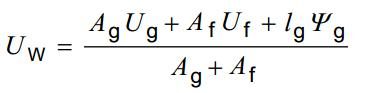Where:
Ag - the area of ​​the light-penetrating part of the window;
Af - frame area (projection onto a vertical plane);
lg - the length of the perimeter of the light-penetrating part of the window;
Yg - linear heat transfer (at the junction between the frame and the light-penetrating part of the window).

## Heat transfer of a plastic window

The international standard ISO 10077-1 gives the minimum value of PVC window frames heat transfer coefficients with two cameras and three cameras. These minimum rates of heat transfer frames of PVC windows - metal-plastic windows – They are, respectively, 2,2 and 2,0 W / m2·TO.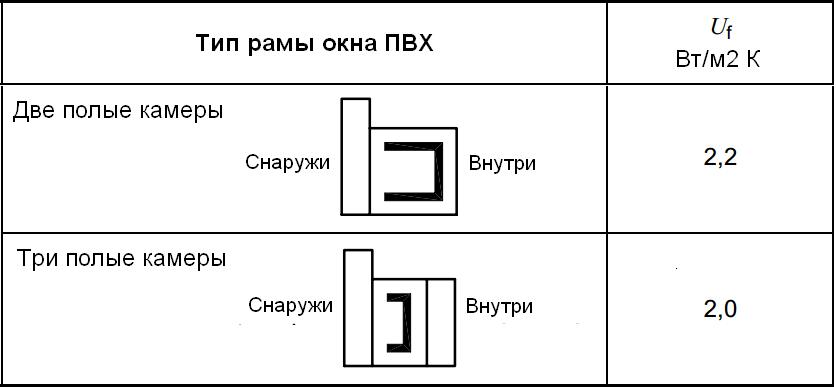Figure 2 - Minimum heat transfer coefficient PVC windows

Typically, PVC window frames are exactly 3 camera. There are PVC frame with 4 and even 5-Tew cameras, but they are more expensive than usual. Standard ISO 10077-1 points, that chamber PVC windows can be considered only a cavity with a minimum width 5 mm. Data frame heat transfer coefficient of such "exotic" PVC windows standard does not.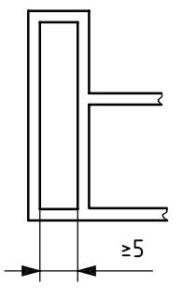Figure 3 - Minimum width of the PVC window frame camera

## Heat transfer timber window

The figure 4 is a plot of the minimum heat transfer coefficient of the wooden window frame, At first, the type of wood (soft or hard) and, Secondly, the thickness of the frame.Figure 4 - coefficient of heat transfer of wooden frames
1 – hard rocks (700 kg / cubic. m and 0,18 W / m K);
2 -soft rocks (500 kg / cubic. m and 0,13 W / m K)

For a typical thickness of the wooden window 50 mm frame is the heat transfer coefficient for softwood 2,0 W / m2 K, but for hardwood - 2,2 W / m2 K. With increasing thickness of the frame 150 mm frame transfer coefficient approaches unity.

## Heat transfer aluminum frame

### Thermorupture aluminum frame

The figure 5 shows the basic structural characteristics of an aluminum frame with termorazvyazkoy as polyamide inserts.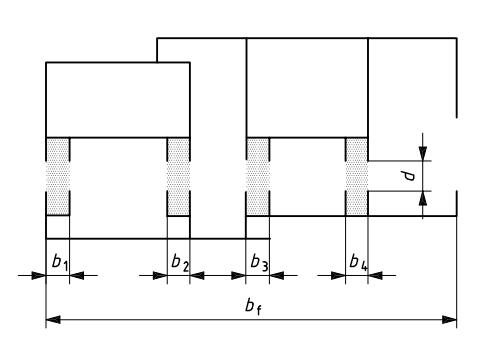Figure 5 - Aluminum frame with polyamide inserts:
0,2 < l ≤ 0,3 W / (m2 TO)
b1 + b2 + b3 + b4 ≤ 0,2 bf

The heat transfer coefficient of a window frame made of thermal break aluminum profiles depends on:

• thermal conductivity coefficient of thermal break material;
• length termorazryva, d, that is, the minimum distance between the outer and inner aluminum profiles;
• width termorazryva, b1+b2+b3+b4;
• the ratio of the total thermal break width (b1+b2+b3+b4) to frame width bf.

### The length of the thermal break

Manufacturers of aluminum windows usually declare the length (or width) of polyamide inserts, which form thermorupture in aluminum profiles of the frame. However, these polyamide inserts have terminations in aluminum profiles at least 2,5 mm on each side. therefore, if used polyamide inserts, for example, length 34 mm, they provide an effective thermal break in the best of all long 29 mm.

### formula

The formula for calculating the heat transfer coefficient of an aluminum window frame is as follows: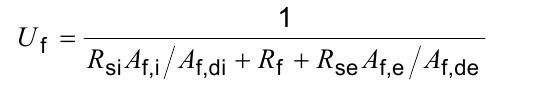Where
Af,i /Af, di Is the ratio of the projection area of ​​the inner surface of the frame onto the plane of the window to the full inner surface of the frame (figure 6);
Af,e /Af,de - the ratio of the projection area of ​​the outer surface of the frame onto the plane of the window to the full outer surface of the frame (figure 6);
Rand – resistance to heat transfer of the inner surface of the frame (air interlayers on the inner surface of the frame), (m2 · K) / W;
RI know – resistance to heat transfer of the outer surface of the frame (air interlayers on the outer surface of the frame), (m2· K) / W;
Rf – heat transfer resistance of the frame section, (m2· K) / W..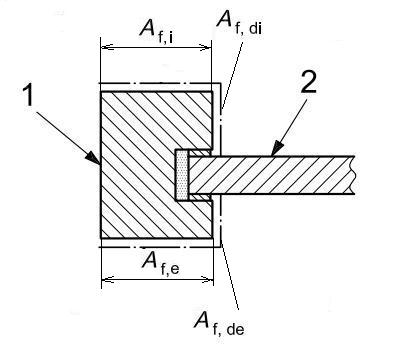Figure 6 - Options form aluminum frame,
which affect the value of its heat COEFFICIENT

### Thermal resistance aluminum frame

The resistance of the frame of an aluminum window without thermal break is taken to be zero: Rf = 0.

Minimal resistance to the aluminum frame, depending on the thermal break length d taken on a continuous graph line in the figure 7.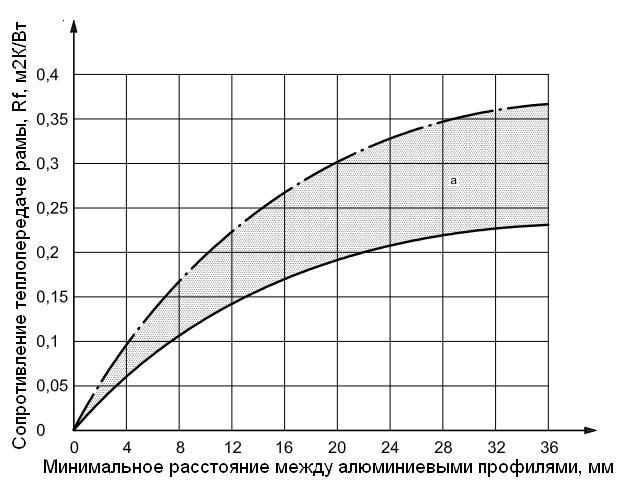Figure 7 - Values ​​of Rf for an aluminum frame with thermal

The shaded area in the figure 7 above the solid line corresponds to the values ​​of resistance to heat transfer of the frame, obtained for different aluminum windows under various conditions in various European countries. Therefore, the top line to be understood as a practical maximum thermal resistance aluminum frames to predetermined values ​​termorazryva d.

## The outer and inner surface of the frame

the resistance value of heat transfer surfaces of the inner and outer vertical window frames by ISO 10077-1 accepted:

• Rand = 0,13 m2· K / W (for the inner surface of the frame);
• RI know = 0,04 m2K / W (for the outer surface of the frame).

## Heat aluminum frame

When a predetermined length of the maximum value of a thermal d aluminum frame heat transfer coefficient is achieved with the formula Ai = Aid and Ae = Aed (cm. Figure 6).

In this case

Thef = 1/ (0,13 + Rf + 0,04) = 1/(Rf + 0,17)

### Aluminum frame without termorazryva

For aluminum frame with no thermal break is taken Rf = 0, what gives

Thef = 1/(0 + 0,17) = 5,9 W / m2·TO

### Aluminum frame with thermal d = 19 mm

For polyamide thermo-insertion 24 mm

1) The minimum value of the resistance to heat transfer of the aluminum frame (along the solid line of the graph of the figure 7):

Rf = 0,18 m2· K / W

2) The maximum value of the resistance to heat transfer of the aluminum frame (along the dotted line of the graph of the figure 7):

Rf = 0,30 m2· K / W

3) Maximum (worst) heat transfer coefficient of the frame with d = 19 mm:

Thef = 1/(0,18 + 0,17) = 1/0,35 = 2,9 W / m2 TO.

4) Minimum (best) heat transfer coefficient of the frame with d = 19 mm:

Thef = 1/(0,30 + 0,17) = 1/0,47 = 2,1 W / m2 TO.

### Aluminum frame with thermal d = 28 mm

For termovstavky 33 mm

1) The minimum value of the resistance to heat transfer of the aluminum frame (along the solid line of the graph of the figure 7):

Rf = 0,22 m2· K / W

2) The maximum value of the resistance to heat transfer of the aluminum frame (along the dotted line of the graph of the figure 7):

Rf = 0,35 m2· K / W

3) Maximum (worst) heat transfer coefficient of the frame with d = 28 mm:

Thef = 1/(0,22 + 0,17) = 1/0,39 = 2,6 W / m2 TO.

4) Minimum (best) heat transfer coefficient of the frame with d = 28 mm:

Thef = 1/(0,35 + 0,17) = 1/0,52 = 1,9 W / m2 TO.

## heat transfer coefficient of aluminum windows

Based on the known heat transfer coefficient of the aluminum frame and the known heat transfer coefficient of the insulating glass unit (table 1) according to the corresponding tables, the minimum heat transfer coefficient of the entire window is determined.

Table 1 - Coefficients of heat transfer of double-glazed windows (fragment)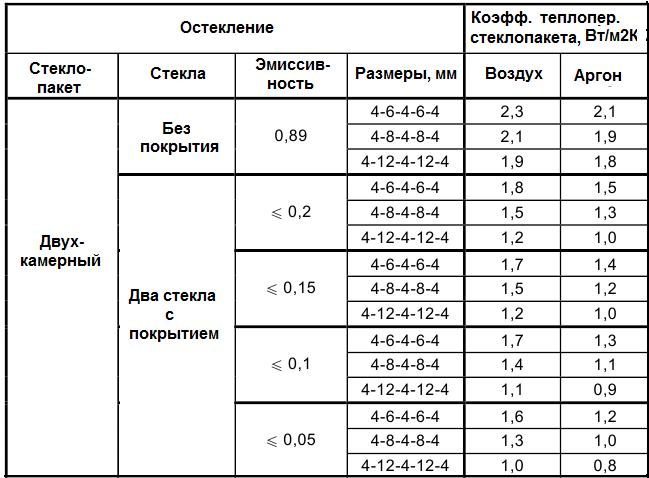Standard ISO 10077-1 It gives four tables to determine the heat transfer coefficient Okan depending on the ratio of the frame area to the total area of ​​the window - 20 and 30 %, and for any type of spacers of insulating glass - conventional and with improved thermal characteristics.

Table 2 - heat transfer coefficients of windows with a ratio of the area of ​​the frame 20 % from the total area of ​​the window (double-glazed windows with conventional spacers) – aluminum frames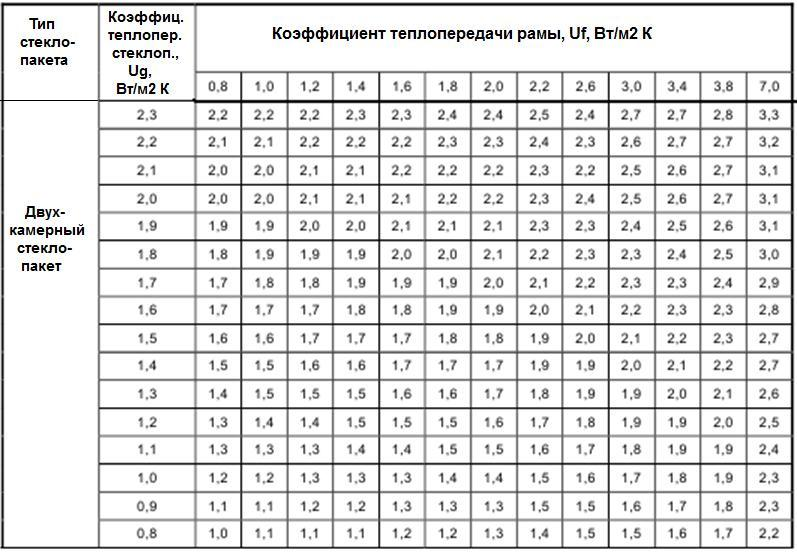Table 3 - heat transfer coefficients of windows with a ratio of the area of ​​the frame 30 % from the total area of ​​the window (double-glazed windows with conventional spacers) – plastic and wooden frames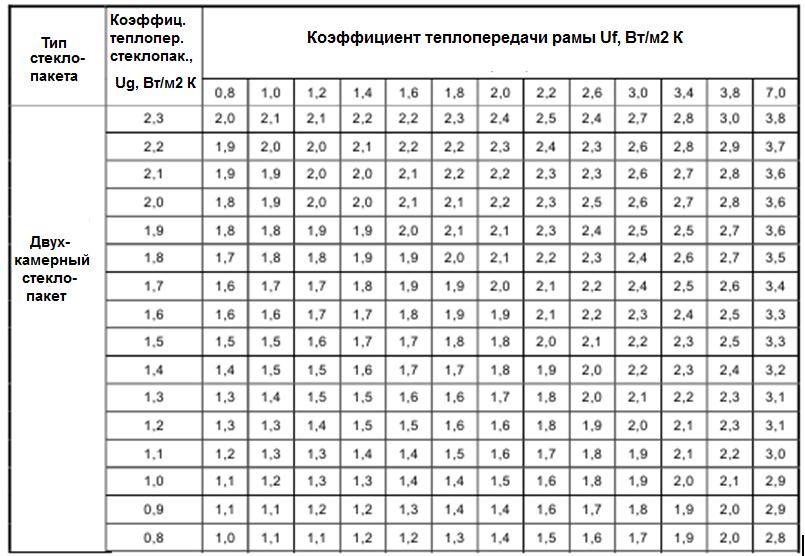1. Wooden window frames have the lowest (best) heat transfer coefficient. When the thickness of the wooden frame of the frame 50 mm frame heat transfer coefficient is about 2,0 W / m2·TO. By increasing the thickness of the wooden frame to 100 mm frame heat transfer coefficient is reduced to 1,5 W / m2·TO, and the 150 mm – to 1,0 W / m2·TO.
2. Top aluminum windows capable of providing heat transfer coefficient to 1,9 W / m2·TO. “worse” three-chambered plastic windows have a frame with a heat transfer coefficient of about 2,2 W / m2·TO. I.e, worst plastic windows can be worse than the best of aluminum windows.
3. Higher strength aluminum alloys as compared with plastics timber and allows to reduce the width of the window frame. The proportion of the frame area of ​​a typical aluminum window is about 20 %, whereas plastic and wooden windows – near 30 %.
Since heat transfer coefficient is always below the glass good, heat transfer coefficient than any frame, it gives the aluminum windows of opportunity to compete with other types of windows, first of all, with plastic, thermal effectiveness. remains, true, the issue of possible condensation on the aluminum frame.